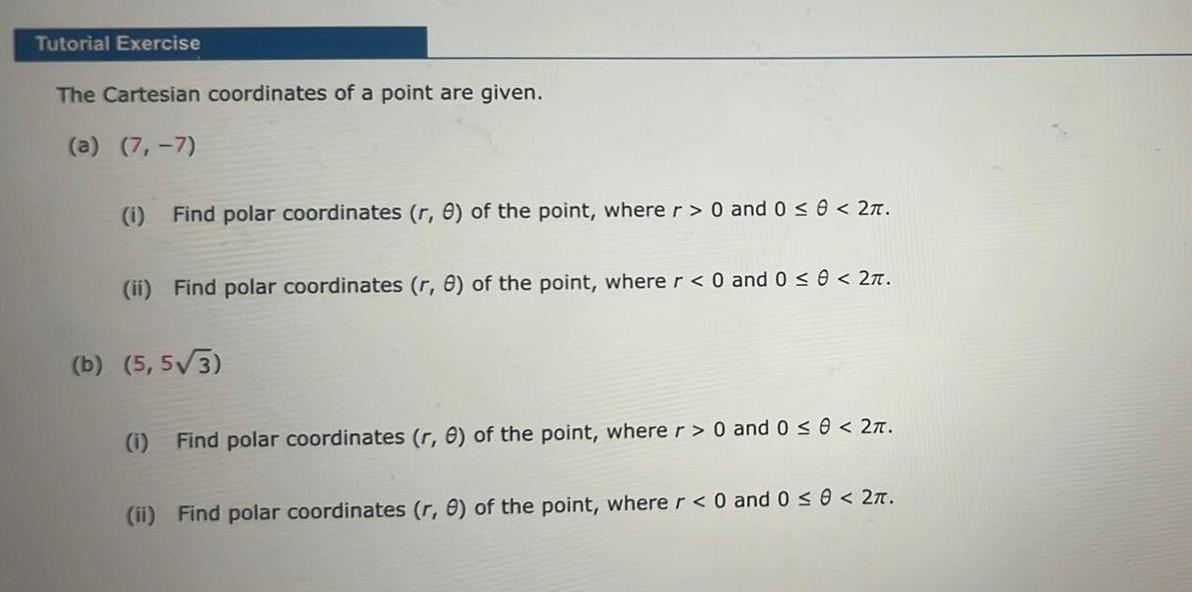Question:

# Tutorial Exercise The Cartesian coordinates of a point are

Last updated: 7/22/2023Tutorial Exercise The Cartesian coordinates of a point are given a 7 7 1 Find polar coordinates r 8 of the point where r 0 and 0 0 2 ii Find polar coordinates r 8 of the point where r 0 and 0 0 2 b 5 5 3 i Find polar coordinates r e of the point where r 0 and 0 0 2 ii Find polar coordinates r e of the point where r 0 and 0 0 2